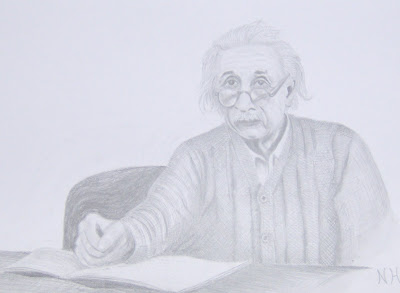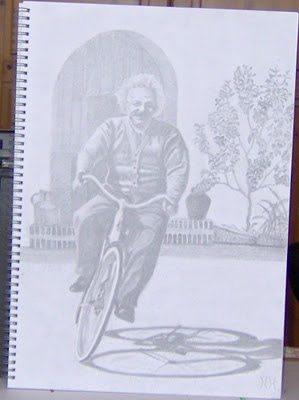Sunday, 19 December 2010

Could we be the dice players of quantum physics?

~

This theory is based on two simple postulates,

1) The first is that the quantum wave particle function explained by Schrödinger’s wave equation represents the forward passage of time itself photon by photon or quanta by quanta.

2) Therefore Heisenberg’s Uncertainty Principle that is formed by the wave function is the same uncertainty that we have with any future event.
~

This can sound odd because we think of “time” in different ways; we have time as a measurement to get from A to B. We also have cosmological time looking back at the history of our Universe as a whole.

Modern physics has no understanding of why we have an arrow of time or a future and a past. But time in this theory is a continuous process forming the future geometry of spacetime photon by photon or moment by moment.

In Quantum Atom Theory the atoms interact with light wave of EMR continuously collapsing the quantum wave particle function forming photons that form electrical charge that in turn form electromagnetic fields in three dimensions.

This forms the local spacetime geometry a time-varying electric field act as a source of a magnetic field, and a time-varying magnetic field was the source of an electric field. When either field is changing in time, then a field of the other is induced.

This will be relative to the position and momentum of the objects creating the time variations, the atoms themselves.

There is a complete and perfect symmetry between positive and negative electric charge. Every particle variety there exist an antiparticle counterpart that forms a profound symmetry within the spacetime geometry of our Universe.

In this theory the continuous formation and breaking of these symmetries relate not just to space but represent the forward flow or passage of time itself.

In modern physics everything has its own reference frames that will have its own proper time relative to its energy of mass. The greater the mass the slower time runs in that reference frame forming Einstein curvature of spacetime.

We are all at the centre of our own reference frame and have our own proper time. I could set up a wave function of where I might be tomorrow and it would have the same uncertainty and probability as the wave function of quantum physics.

Because of quantum probability we cannot predict when an individual nucleus will decay in a radioactive sample. Therefore we have the half-life, the time it takes half the nuclei in a sample to decay over a period of time.

This is the same probability we have in classical physics if we take a group of people we cannot predict the life of an individual person of the group, but we can calculate a half-life for the whole group statistically over a period of time.

This theory might sound child-like but it is based on the Correspondence Principle and has a one-to-one correspondence between an element of the theory and an element of reality.

We have probability propagating according to the laws of causality. This gives us a coherent physical interpretation of quantum physics. Objects will form their own spacetime geometry relative to their energy or mass. They will also form the uncertainty of their own future potential as time unfolds ∆E ∆t ≥ h/2π.

The Planck constant h is a constant of action within the geometry of spacetime forming part of a physical process that forms an independent objective reality that we can interact with forming the possible into the actual.

We see and feel this as the forward passage of time and as the uncertainty and probability of everyday life. We see a temporary image of our Universe moment by moment continuously changing made up of an infinite number of photons.
~

Each electron has its own three-dimensional spacetime two electrons requires six-dimensions three electrons require nine dimensions and so on.

But no two electrons can share the same quantum state at the same time. This is just the same as an object in our everyday life cannot be in two places at the same time.

Because classical mechanics is an approximation of quantum mechanics we only ever have three-dimensions and the other dimensions represent a potential sequence forming the time continuum.

The deep fundamental difference between quantum and classical mechanics is that quantum mechanics represents the forward passage of time itself.

I hope to base this theory on pure mathematics and therefore pure physics. I believe this can be done in just three dimensions and one variable time.
~Each photon electron coupling represents zero representing a new moment it time (the moment of now) with the positive numbers marching off towards an infinite future, their negative sibling recede towards a limitless past (the positive and negative of EMW).

In this theory it is because time only moves forward that matrix mathematics of Quantum Mechanics does not obey the commutative law of multiplication in which x times y is always equal to y times x nothing can go backwards in time!

Add to this that the probability of the wave function only works one way in time. It is within the rules of quantum physics to calculate backwards and work out what the position and momentum was at some time in the past exactly as we can in our everyday life.

It makes no difference if you use Schrödinger’s wave equation or the matrix algebra of Heisenberg’s quantum particles. This theory gives us an intuitive picture capturing the existence of an underlying layer of reality. This gives us an objective reality to quantum mechanics as a process of continuous symmetry forming and breaking a process of continuous creation.
~Einstein forming his own position and momentum, therefore is own probability.

~

I am an artist and therefore outside of the scientific community because of this there is no peer review and any help promoting this theory in the scientific community will be gratefully welcomed.
~

~

Sunday, 5 December 2010

Einstein's dream an objective quantum mechanicsEinstein’s worked until the end of his life to find an independent objective reality to quantum mechanics.

Einstein believed the quantum theory was correct but not complete. He believed in a hidden variable that would give quantum mechanics an objective reality that would fit in with the reality of our everyday life.

Most other physicist believed that no reasonable definition of reality could be expected to permit the paradoxes of quantum mechanics.

Einstein said “the next stage in the development of theoretical physics will bring us a theory of light that may be conceived of as a sort of fusion of wave and of emission theory of light.
~In Einstein’s Universe position and momentum at the quantum level would be explained in just the same way as it is for an object in our everyday life.

Einstein said “while we have thus shown that the wave function does not provide a complete description of the physical reality, we left open the question of whether or not such a description exists. We believe, however, that such a theory is possible”.

Quantum Atom theory is such a theory and from two simple postulates can explain the paradoxes of quantum mechanics.

The first postulate is that the wave function explained mathematically by Schrodinger’s wave equation represents the arrow of time forming the geometry of spacetime.

The second postulate is that Heisenberg’s Uncertainty Principle that is formed by the expanding wave function is the same uncertainty that we have with any future event.

Without Heisenberg’s Uncertainty Principle we would not have the uncertainty and probability of everyday life. The probability of the quantum wave particle function only works one way in time. Just like the uncertainty of everyday life it is always possible to calculate backwards and work out exactly what the position and momentum was at some time in the past just like looking back in history

In the General Theory of Relativity Einstein beautifully explained that the presence of objects in space curves it forming the curvature or geometry of spacetime. If the object moves around then the curvature of space will change as well.

This theory explains the process of how objects form their own rate of time forming the curvature of spacetime. An object will react with the wave particle duality of the light distorting the geometry of spacetime relative to their energy or mass. This will form the passage of time photon by photon the greater the energy or mass the slower time will run in that reference frame.

A moving object will distort its own spacetime geometry more in the direction of its motion. This will form an unbalanced force and the object will keep moving in that direction unless acted upon by an external object. Therefore we have Galileo’s principle of inertia supporting Newton’s first law of motion.

In this theory we can all form our own spacetime geometry forming our own future because our actions will change our energy or mass.

This is why the Planck Constant is a constant of action and represents part of the continuum of time continuously coming into existence photon by photon or moment by moment.
~
I am an artist and therefore outside of the scientific community because of this there is no peer review and any help promoting this theory in the scientific community will be gratefully welcomed.
~